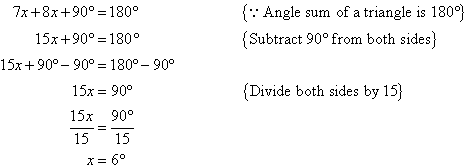Year 7 Interactive Maths - Second Edition

## Angle Sum of a Triangle

### Activity 2

###### Step 1:

Draw a triangle ABC and cut out the three angles.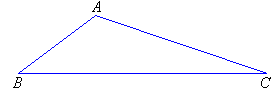###### Step 2:

Rearrange the three angles to form a straight angle on a straight line.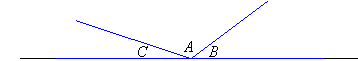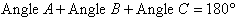So, the angle sum of a triangle is 180°.

To investigate if this works for all triangles, repeat the above process for four different triangles.

Copy and complete the following statements:
a.  The three angles of a triangle can be arranged to form a ……….. angle.
b.  The sum of three angles in a triangle is ………..

### Finding the Third Angle of a Triangle

If the measurements of two angles of a triangle are known, then the third angle can be calculated.

#### Example 3

Calculate the size of the missing angle in the following triangle.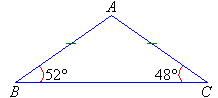##### Solution:

Let the missing angle be x.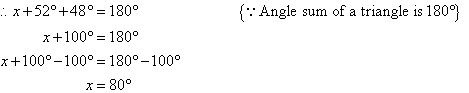So, the missing angle is 80°.

#### Example 4

Find the values of the pronumerals x and y in the following diagram: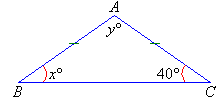##### Solution: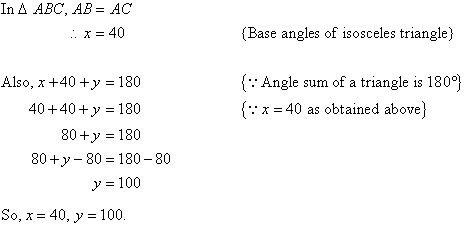#### Example 5

Find the value of the pronumeral x in the following diagram: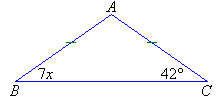##### Solution: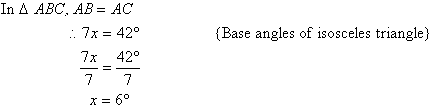#### Example 6

Find the value of the pronumeral x in the following diagram: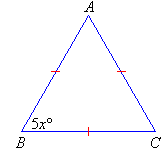##### Solution: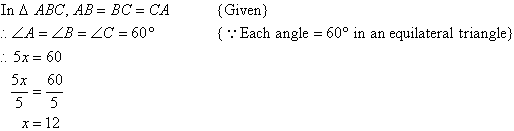#### Example 7

Find the values of the pronumerals x and y in the following diagram: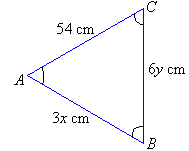##### Solution: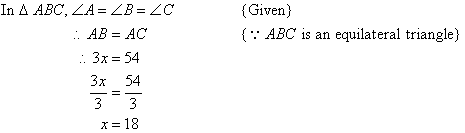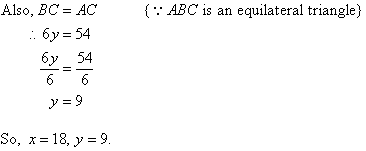#### Example 8

Find the value of the pronumeral x in the following diagram: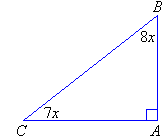##### Solution: